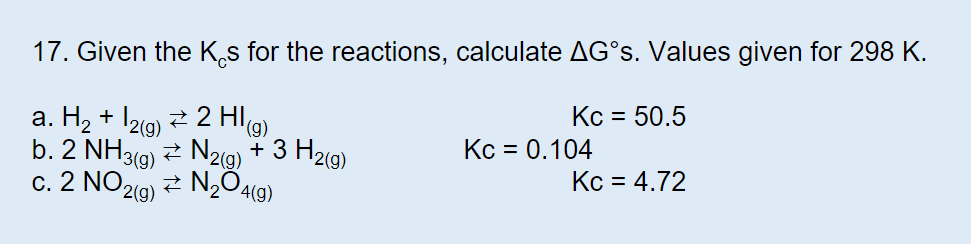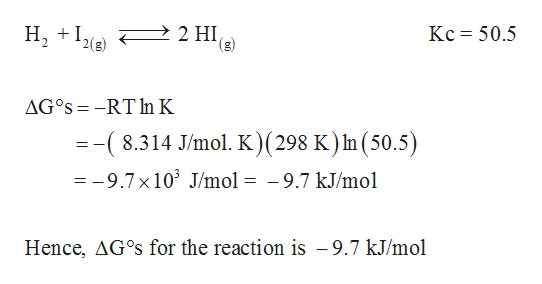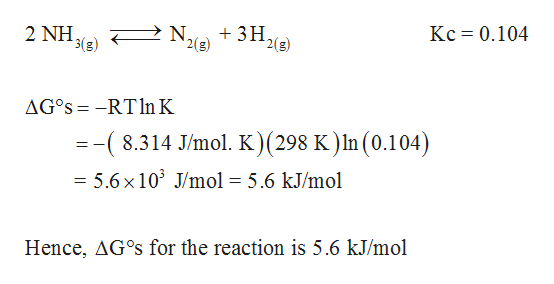# 17. Given the Ks for the reactions, calculate AG°S. Values given for 298 K.Kc 50.5a. H2+ 12g)2 H)b. 2 NH3(g)N2(9) + 3 H2(g)c. 2 NO 2(g)Kc 0.104Kc 4.72N204(g)

Question
3 viewshelp_outlineImage Transcriptionclose17. Given the Ks for the reactions, calculate AG°S. Values given for 298 K. Kc 50.5 a. H2+ 12g)2 H) b. 2 NH3(g)N2(9) + 3 H2(g) c. 2 NO 2(g) Kc 0.104 Kc 4.72 N204(g) fullscreen
check_circle

Step 1

(a)

ΔGos can be calculated as follows,help_outlineImage Transcriptionclose2 HI (g) H, Kc 50.5 2(g) AG°S RT In K =-(8.314 J/mo. K)(298 K) In (50.5) =-9.7x 10 J/mol = -9.7 kJ/mol Hence, AG°S for the reaction is - 9.7 kJ/mol fullscreen
Step 2

(b)

ΔGos can be calculate...help_outlineImage TranscriptioncloseKc 0.104 2 ΝΗ, 3(g) 3H2 '2(g) 2 NH N, AG°S -RTln K =-(8.314 J/mol. K)(298 K ) In (0.104) = 5.6x 103 J/mol = 5.6 kJ/mol Hence, AG°s for the reaction is 5.6 kJ/mol fullscreen

### Want to see the full answer?

See Solution

#### Want to see this answer and more?

Solutions are written by subject experts who are available 24/7. Questions are typically answered within 1 hour.*

See Solution
*Response times may vary by subject and question.
Tagged in

### Chemical Thermodynamics